# Book 1 Review

You will now practice all the skills you learned in Book 1. Check your work using the answer key at the end of the review.

If you can’t remember how to do a question, go back to the lesson on this topic to refresh your memory. The unit and topic for where each question came from is listed next to the question.

Example: 1-B means Unit 1, Topic B

# 1-A

1. Count the number of things in each picture. Write the number and word name.
1.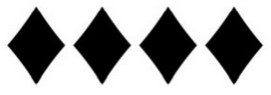Numeral:

Word Name:

1.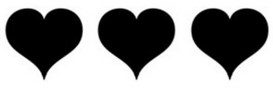Numeral:

Word Name:

1.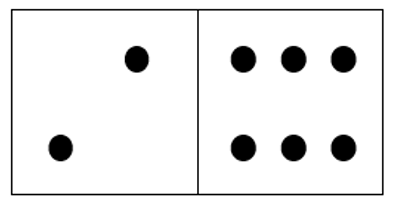Numeral:

Word Name:

1.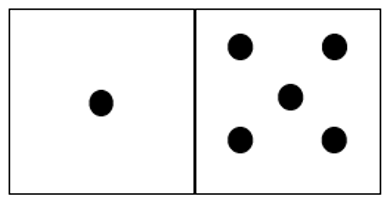Numeral:

Word Name:

# 1-B

1. Fill in the blanks to make each sentence true. Draw a picture for B and D.
1. 58 means           tens and           ones.
2. 18 means           tens and           ones.
3. 471 means           hundreds,           tens and           ones.
4. 127 means           hundreds,           tens and           ones.
2. Write the place value names (ones, tens, hundreds) for each underlined digit.
1. 56
2. 239
3. 98
4. 534
3. Name the digit for the place value named from the number 5782.
1. Ten
2. Hundreds
4. Write the word names for the numbers.
1. 17
2. 342
3. 625
5. Write numerals for these word names.
1. seventy-five
2. nineteen
3. seven hundred fifty
4. nine hundred five
5. eight hundred seventy-three

# 1-C

1. Place a box around the larger number.
1. 452, 245
2. 678, 687
2. H. Arrange these numbers in order from smallest to largest.
1. 86, 668, 886, 686, 868, 66, 866
2. 23, 323, 223, 33, 332, 322, 232
3. Write <, > or = in each blank as needed.
1. 23           34
2. 118           118
3. 667           576
4. 405           450

# 1-D

1. Round each number to the nearest ten.
1. 52
2. 123
3. 178
4. 89
2. Word problems. For each problem, round the numbers to the nearest 10.
1. The polar bear can weigh 1 002 kilograms, a koala bear can weigh 14 kilograms, a panda bear can weigh 113 kilograms, a Kodiak bear can weigh 679 kilograms and a black bear can weigh 272 kilograms. Round each number to the nearest 10.
Bear Number Rounded Number
Polar Bear
Koala Bear
Panda Bear
Kodiak Bear
Black Bear
3. How much money do you have?
1.           cents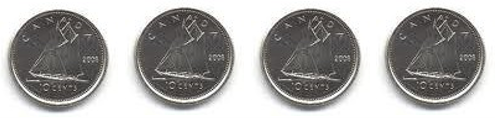2.           dollars# 2-A

1. \begin{align}0\\+8\\ \hline \\ \end{align}
2. \begin{align}2\\+3\\ \hline \\ \end{align}
3. \begin{align}8\\+2\\ \hline \\ \end{align}
4. \begin{align}1\\+4\\ \hline \\ \end{align}
5. \begin{align}5\\+0\\ \hline \\ \end{align}
6. \begin{align}9\\+5\\ \hline \\ \end{align}
7. \begin{align}6\\+7\\ \hline \\ \end{align}
8. \begin{align}3\\+6\\ \hline \\ \end{align}
1. 7 + 4 =
2. 3 + 0 =
3. 2 + 9 =
4. 9 + 8 =
5. 6 + 2 =
6. 5 + 6 =
7. 8 + 9 =
8. 4 + 2 =
3. Find the sums.
1. \begin{align}4\\5\\+3\\ \hline \\ \end{align}
2. \begin{align}2\\7\\+8\\ \hline \\ \end{align}
3. \begin{align}4\\2\\+8\\ \hline \\ \end{align}
4. \begin{align}4\\6\\+7\\ \hline \\ \end{align}
5. \begin{align}3\\2\\+3\\ \hline \\ \end{align}
6. \begin{align}6\\1\\+5\\ \hline \\ \end{align}
4. Find the sums.
1. \begin{align}5\\2\\3\\+4\\ \hline \\ \end{align}
2. \begin{align}2\\3\\4\\+7\\ \hline \\ \end{align}
3. \begin{align}3\\0\\1\\+2\\ \hline \\ \end{align}
4. \begin{align}2\\3\\1\\+2\\ \hline \\ \end{align}
5. \begin{align}5\\1\\3\\+2\\ \hline \\ \end{align}
6. \begin{align}4\\3\\2\\+6\\ \hline \\ \end{align}
5. Find the perimeter of the shape. Be sure to put the unit of measure in your answer. Write the name of the shape below the picture.
1.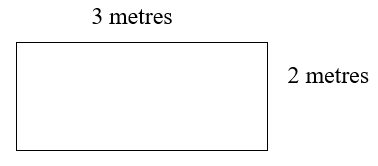2.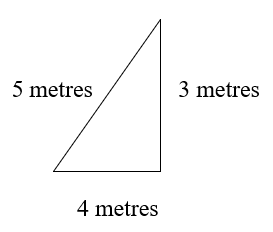3.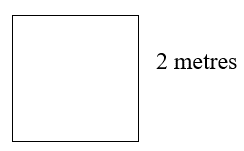6. Find the sums.
1. \begin{align}46\\+33\\ \hline \\ \end{align}
2. \begin{align}35\\+93\\ \hline \\ \end{align}
3. \begin{align}82\\+56\\ \hline \\ \end{align}
4. \begin{align}91\\+17\\ \hline \\ \end{align}
5. \begin{align}740\\+859\\ \hline \\ \end{align}
6. \begin{align}638\\+610\\ \hline \\ \end{align}
7. Word problems.
1. Seven cars were in the first row. Four cars were in the second row. How many cars are there in the first two rows?
2. One bicycle stored ordered 56 bikes. Another store ordered 72 bikes. How many bikes did both stores order?
3. A mail carrier walked 51 kilometres in a week. The next week she walked 48 kilometres the next week. How far did she walk in two weeks?

# 3-A

1. Check out your subtraction facts.
1. \begin{align}9\\-5\\ \hline \\ \end{align}
2. \begin{align}6\\-3\\ \hline \\ \end{align}
3. \begin{align}17\\-8\\ \hline \\ \end{align}
4. \begin{align}14\\-7\\ \hline \\ \end{align}
5. \begin{align}14\\-9\\ \hline \\ \end{align}
6. \begin{align}11\\-2\\ \hline \\ \end{align}
7. \begin{align}12\\-5\\ \hline \\ \end{align}
8. \begin{align}9\\-3\\ \hline \\ \end{align}
2. Subtract across or horizontally
1. 4 − 1 =
2. 8 − 2 =
3. 17 − 8 =
4. 11 − 6 =
5. 6 − 4 =
6. 11 − 3 =

# 3-B

1. Find the differences.
1. \begin{align}76\\-25\\ \hline \\ \end{align}
2. \begin{align}84\\-43\\ \hline \\ \end{align}
3. \begin{align}95\\-74\\ \hline \\ \end{align}
4. \begin{align}69\\-16\\ \hline \\ \end{align}
5. \begin{align}852\\-321\\ \hline \\ \end{align}
6. \begin{align}789\\-650\\ \hline \\ \end{align}
2. Word problems. Solve each work problem.
1. There were 18 roses in a bouquet. Milton gave 9 roses away. How many roses were left?
2. A city has 89 mail carriers. One day only 54 were at work. How many were not at work?
3. Mariko and Stefan went 5-pin bowling. Mariko scored 274 points while Stefan scored 152. How many more points did Mariko score?

# 4-A

1. Estimate the following answers. Be sure to round to the nearest 10 before adding.
1. \begin{align}81\\+74\\ \hline \\ \end{align}
2. \begin{align}53\\+39\\ \hline \\ \end{align}
3. \begin{align}43\\+68\\ \hline \\ \end{align}
4. \begin{align}733\\+719\\ \hline \\ \end{align}
5. \begin{align}907\\+448\\ \hline \\ \end{align}
6. \begin{align}623\\+914\\ \hline \\ \end{align}
2. Estimate the following answers. Be sure to round to the nearest 10 before subtracting.
1. \begin{align}82\\-59\\ \hline \\ \end{align}
2. \begin{align}67\\-38\\ \hline \\ \end{align}
3. \begin{align}61\\-17\\ \hline \\ \end{align}
4. \begin{align}968\\-426\\ \hline \\ \end{align}
5. \begin{align}577\\-171\\ \hline \\ \end{align}
6. \begin{align}742\\-533\\ \hline \\ \end{align}
3. Word problems. Estimate the following answers. Be sure to round to the nearest 10 before adding or subtracting.
1. Mr. Han worked in his store for 33 years. Before owning a store, he had worked in a bank for 24 years. How many years has Mr. Han worked?
2. The longest span of the Lions Gate Bridge in Vancouver is 473 metres. The longest span of the Confederation Bridge in Prince Edward Island is 247 metres. What is the difference?

# 4-B

1. Write the following times in standard format.
1. 3 h, 22 min, 51 s
2. 8 h, 38 min, 9 s
3. 10 h, 18 min, 23 s
4. 7 h, 43 min, 34 s
2. Write the following times using a.m. or p.m.
1. The movie begins at 8:30 in the evening.
2. The coffee shop opens at 5:15 in the morning.
3. The shopping mall closes at 10:00 at night.
3. Round the following times to the nearest hour.
1. The running time for the movie was 2 hours and 25 minutes.
2. It took 5 hours and 53 minutes to go the hockey and return home after the game.
4. The following things give the idea of a shape. Write the name of the shape in each blank.
1. A tree is a          .
2. A swimming pool is a          .
3. A quarter is a          .
5. What shape are the following things? Write triangle, square, rectangle or circle.
1.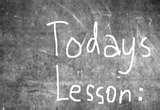2.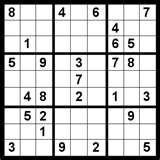# Answers to Book 1 Review

1. 4, four
2. 3, three
3. 8, eight
4. 6, six
1. 5 tens, 8 ones
2. 1 ten, 8 ones
3. 4 hundreds, 7 tens, 1 one
4. 1 hundred, 2 tens, 7 ones
1. tens
2. ones
3. tens
4. hundreds
1. 8
2. 7
1. seventeen
2. three hundred forty-two
3. six hundred twenty-five
1. 75
2. 19
3. 750
4. 905
5. 873
1. 452
2. 687
1. 66, 86, 668, 686, 866, 868, 886
2. 23, 33, 223, 232, 322, 323, 332
1. <
2. =
3. >
4. <
1. 50
2. 120
3. 180
4. 90
1. Bear Number Rounded Number
Polar Bear 1002 1000
Koala Bear 14 10
Panda Bear 113 110
Kodiak Bear 679 680
Black Bear 272 270
1. 40 cents
2. 12 dollars
1. 8
2. 5
3. 10
4. 5
5. 5
6. 14
7. 13
8. 9
1. 11
2. 3
3. 11
4. 17
5. 8
6. 11
7. 17
8. 6
1. 12
2. 17
3. 14
4. 17
5. 8
6. 12
1. 14
2. 16
3. 6
4. 8
5. 11
6. 15
1. 10 metres, rectangle
2. 12 metres, triangle
3. 8 metres, square
1. 79
2. 128
3. 138
4. 108
5. 1599
6. 1248
1. 11 cars
2. 128 bikes
3. 99 kilometres
1. 4
2. 3
3. 9
4. 7
5. 5
6. 9
7. 7
8. 6
1. 3
2. 6
3. 9
4. 5
5. 2
6. 8
1. 51
2. 41
3. 21
4. 53
5. 531
6. 139
1. 9 roses
2. 35 mail carriers
3. 122 points
1. 80 + 70 = 150
2. 50 + 40 = 90
3. 40 + 70 = 110
4. 730 + 720 = 1450
5. 910 + 450 = 1360
6. 620 + 910 = 1530
1. 80 − 60 = 20
2. 70 − 40 = 30
3. 60 − 20 = 40
4. 970 − 430 = 540
5. 580 − 170 = 410
6. 740 − 530 = 210
1. 50 years
2. 220 metres
1. 3:22:51
2. 8:38:09
3. 10:18:23
4. 7:43:34
1. 8:30 p.m.
2. 5:15 a.m.
3. 10:00 p.m.
1. 2 hours
2. 6 hours
1. triangle
2. rectangle
3. circle
1. rectangle
2. square